The UCM Procedure

SLOPE Statement

• SLOPE <options>;

The SLOPE statement is used to include a slope component in the model. The slope component cannot be used without the level component (see the LEVEL statement). The level and slope specifications jointly define the trend component of the model. A SLOPE statement without the accompanying LEVEL statement is ignored. The equations of the trend, defined jointly by the level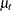and slope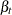, are as follows: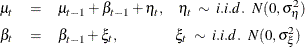The SLOPE statement is used to specify the value of the disturbance variance,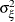, in the slope equation, and to request forecasts of. The following examples illustrate this statement:

      level;
slope;


The preceding statements fit a model with a locally linear trend. The disturbance variances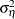andare estimated from the data. You can request a locally linear trend with fixed slope by using the following statements:

      level;
slope variance=0 noest;

NOEST

fixes the value of the disturbance variance,, to the value specified in the VARIANCE= option.

PLOT=FILTER
PLOT=SMOOTH
PLOT=( <FILTER> <SMOOTH> )

requests plotting of the filtered or smoothed estimate of the slope component.

PRINT=FILTER
PRINT=SMOOTH
PRINT=( <FILTER> <SMOOTH> )

requests printing of the filtered or smoothed estimate of the slope component.

VARIANCE=value

specifies an initial value for the disturbance variance,, in theequation at the start of the parameter estimation process. Any nonnegative value, including zero, is an acceptable starting value.